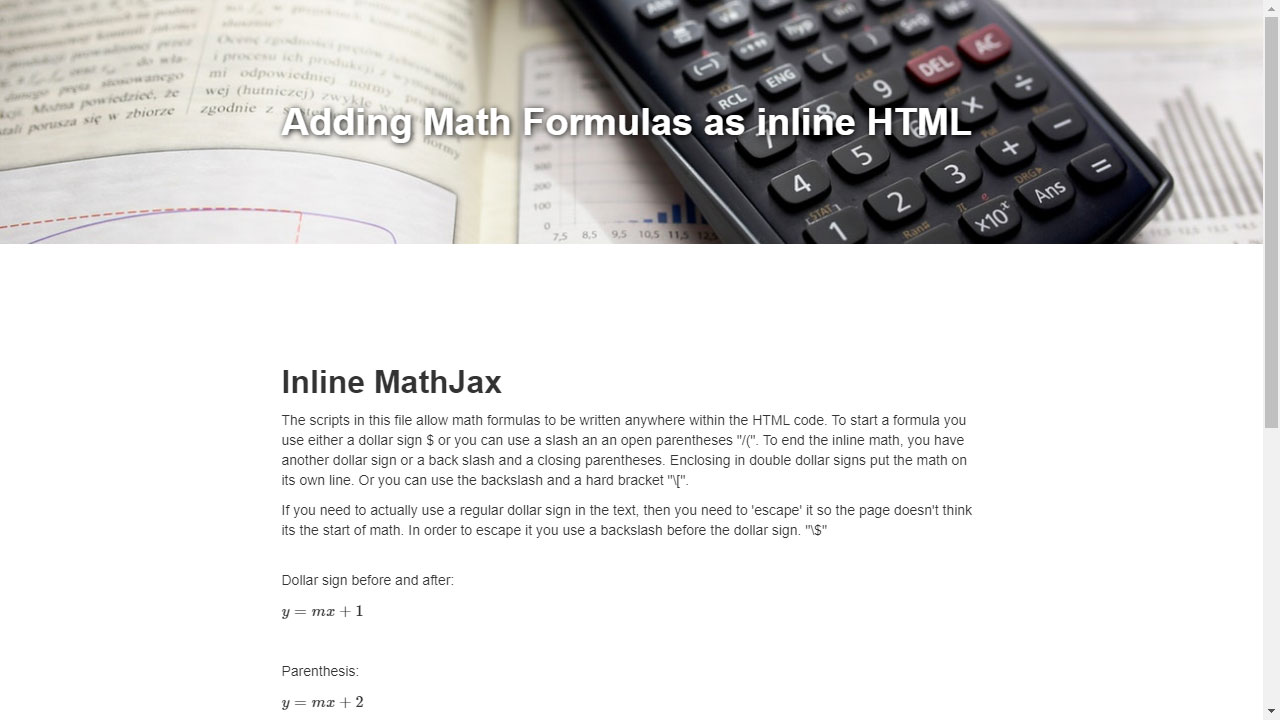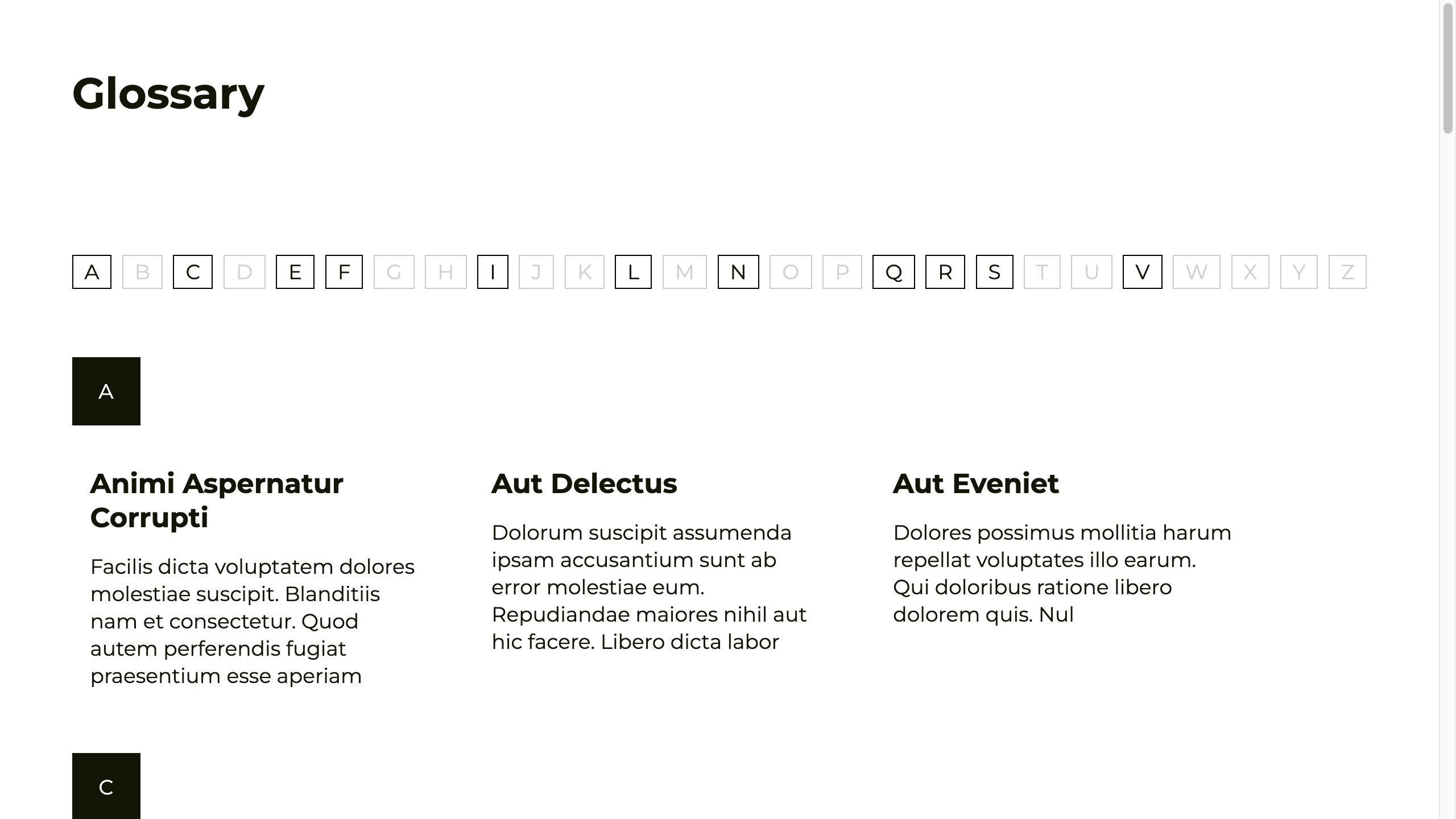Back to all Elements

# Math formulas in CMS

## Writing inline (LaTeX) math formulas anywhere on a static or dynamic page using MathJax.

The script allows math formulas to be written anywhere within the HTML code. To start the inline math you use either a dollar sign $or you can use a slash and an open parentheses "/(". To end the inline math, you have another dollar sign or a back slash and a closing parentheses. Enclosing in double dollar signs put the math on a seperate line. So, let's get to it- First we add the MathJax JS library and the Polyfill.js dependency in our page <script src="https://polyfill.io/v3/polyfill.min.js?features=es6"></script> <script type="text/javascript" id="MathJax-script" async src="https://cdn.jsdelivr.net/npm/mathjax@3/es5/tex-mml-chtml.js"></script> Copy Now we add the MathJax function to initiate the script and define what is a math formula: <script> MathJax = { tex: { inlineMath: [['$', '$'], ['\$$', '\$$']] }, }; </script> Copy If you need to use a dollar sign in the text, you can use a backslash before the dollar sign. "\$"

Dollar sign before and after:

$y=mx+1$

Parenthesis:

$$y=mx+2$$

Double Dollar Signs:

$$y=mx+3$$

Hard Bracket:

$y=mx+5$

In a sentence:
When $a \ne 0$, there are two solutions to $$ax^2 + bx + c = 0$$ and they are

$$x = {-b \pm \sqrt{b^2-4ac} \over 2a}.$$

#### Preview:Share:Whenever possible, use Class Names to target the elements in your interaction. You never know when you'll need to duplicate and use it somewhere else...

### 3d Video Slider (swiper.js)CloneableCMSJavaScript

A 3d slider with video items, plays and pauses on click & slide change.### Webflow Dashboard Workspaces HackWebflow UI HackCodeCSS

Convert the Workspaces dropdown to an open sidebar with custom order.### GlossaryCloneableJavaScriptCMS

A clonable for creating an Alphabetical glossary